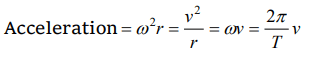## Motion in a Plane Questions and Answers Part-9

1. A cyclist riding the bicycle at a speed of $14\sqrt{3} ms^{-1}$   takes a turn around a circular road of radius $20\sqrt{3} m$   without skidding. Given$g=9.8 ms^{-2}$   , what is his inclination to the vertical
a) $30^{\circ}$
b) $90^{\circ}$
c) $45^{\circ}$
d) $60^{\circ}$

Explanation:2. If a cycle wheel of radius 4 m completes one revolution in two seconds. Then acceleration of a point on the cycle wheel will be
a) $\pi^{2} m/s^{2}$
b) $2\pi^{2} m/s^{2}$
c) $4\pi^{2} m/s^{2}$
d) $8\pi m/s^{2}$

Explanation:3. A bob of mass 10 kg is attached to wire 0.3 m long. Its breaking stress is $4.8\times 10^{7}N\diagup m^{2}$  . The area of cross section of the wire is $10^{-6} m^{2}$. The maximum angular velocity with which it can be rotated in a horizontal circle

Explanation:4. If a cyclist moving with a speed of 4.9 m/s on a level road can take a sharp circular turn of radius 4 m, then coefficient of friction between the cycle tyres and road is
a) 0.41
b) 0.51
c) 0.61
d) 0.71

Explanation:5. A car moves on a circular road. It describes equal angles about the centre in equal intervals of time. Which of the following statement about the velocity of the car is true
a) Magnitude of velocity is not constant
b) Both magnitude and direction of velocity change
c) Velocity is directed towards the centre of the circle
d) Magnitude of velocity is constant but direction changes

Explanation: As body covers equal angle in equal time intervals. its angular velocity and hence magnitude of linear velocity is constant

6. A scooter is going round a circular road of radius 100 m at a speed of 10 m/s. The angular speed of the scooter will be

Explanation:7. A particle of mass M moves with constant speed along a circular path of radius r under the action of a force F. Its speed is
a) $\sqrt{\frac{r F}{m}}$
b) $\sqrt{\frac{F}{r}}$
c) $\sqrt{F m r}$
d) $\sqrt{\frac{F}{m r}}$

Explanation:8. In an atom for the electron to revolve around the nucleus, the necessary centripetal force is obtained from the following force exerted by the nucleus on the electron
a) Nuclear force
b) Gravitational force
c) Magnetic force
d) Electrostatic force

Explanation: Electrostatic force provides necessary centripetal force for circular motion of electron.

9. A particle moves with constant speed v along a circular path of radius r and completes the circle in time T. The acceleration of the particle is
a) $2\pi v\diagup T$
b) $2\pi r\diagup T$
c) $2\pi r^{2}\diagup T$
d) $2\pi v^{2}\diagup T$10. The maximum velocity $\left(ms^{-1}\right)$   with which a car driver must traverse a flat curve of radius 150 m and coefficient of friction 0.6 to avoid skidding is